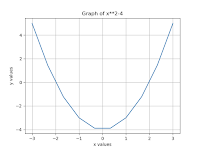# Python to find and plot the root using Bisection Method

In this, you will learn how to find and plot the root of equation using Bisection MethodYou have to learn about some basics concepts of Python Programming.

The list of topics given below:

Introduction to Python

Basics of Python

In this section, we discuss four examples of Bisection Method use to find and plot the root of the given equations.

### Introduction

If the floating number x is given two f (x) and two numbers ‘a’ and ‘b’, then f (a) * f (b) <0 and f (x) are constant in [a, b]. Where f (x) represents an algebraic equation. Find the root of the function at interval [a, b] (or find the value of x which is f (x) 0).

### Bisection Method

This method is also known as interval halving method, binary search method or dichotomy method.

This method is used to find the origin of the equation at a given interval, where the value of ‘x’ is f (x) = 0.

This method is based on the intermediate value theory that if f (x) is a continuous function then a and b are two real numbers f (a) * f (b) 0 and f (b) <0), then there must be at least one root between them.

Now let's take a look at how to write a Program to find the root of the given equation. We discuss four different examples with different equation. These examples are given below:

Example 1:

Write a Program to find the root of equation y = x³-x²+2

#bisection method

`Python Code:def f(x):    y = x**3 - x**2+2    return ya = -200b = 300def bisection(a,b):    if f(a)*f(b)>0:        print("no root found")        return    c = a    while ((b-a)>=0.01):        c = (a +b)/2        if f(c)==0:            break        if f(c)*f(a)<0:            b = c        else:            a = c    print("root of equation is =", c)bisection(a,b)Output:`
```root of equation is = -1.00250244140625
```

Example 2:

#### Program to find and plot the root of equation y= x²-4 which lies in [0,4]

Python Code:

`from pylab import *def f(x):    y = x**2 - 4    return yx = linspace(-3,3,10)xa = 0xb = 4xc = (xa+xb)/2while abs(f(xc))>0.001:    if f(xa)*f(xc)>0:        xa = xc    else:        xb = xc    xc = (xa + xb)/2print("the root of equation is =", xc)plot(x,f(x))xlabel('x values')ylabel('y values')title('Graph of x**2-4')grid(True)show()`
`Output:`
```the root of equation is = 2.0
```
``

#### Example 3:

`Write a Program to find the root of equation y = x5-3x+1 which lies in [0,1]Python code:from pylab import *def f(x):    y = x**5-3*x+1    return yx = linspace(-3,3,10)xa = 0xb = 1xc = (xa+xb)/2while abs(f(xc))>0.001:    if f(xa)*f(xc)>0:        xa = xc    else:        xb = xc    xc = (xa + xb)/2print("the root of equation is =", xc)plot(x,f(x))xlabel('x values')ylabel('y values')title('Graph of x**5-3*x+1')grid(True)show()`
`Output:`
```the root of equation is = 0.3349609375
```Example 4:
`Write a Program to find the root of equation y = x3-10x2+5 which lies in [0,1]Python code:from pylab import *def f(x):    y = x**3-10*x**2+5    return yx = linspace(-3,3,10)xa = 0xb = 1xc = (xa+xb)/2while abs(f(xc))>0.001:    if f(xa)*f(xc)>0:        xa = xc    else:        xb = xc    xc = (xa + xb)/2print("the root of equation is =", xc)plot(x,f(x))xlabel('x values')ylabel('y values')title('Graph of x**3-10*x**2+5')grid(True)show()`
Output:
the root of equation is = 0.734619140625